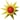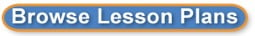Search results for intercepts:
 Browse All Lesson Plans Lesson Plan Name Grades Manipulating Graphs 7 to 8(0 stars, 1 ratings)This lesson will help students make a connection to the slope-intercept form of a graph, y=mx+b and how it relates to the real world. Interpret the equation 8 to 8(0 stars, 1 ratings)To interpret the equation of line, students will rotate through three stations. Each station will require the students to interpret the equation but using different techniques. Quadratics in Nature and Architecture 9 to 12(0 stars, 3 ratings)Students will discover real-life applications of quadratic functions using video cameras. The students will learn how to write equations for the parabolas that they find in real-life.